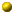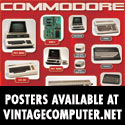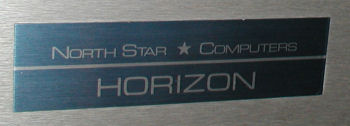Search Posts:

Focal Lunar Lander

SHAREby Bill Degnan - 07/15/2013 10:05
W A
C-FOCAL,1969

01.04 T "CONTROL CALLING LUNAR MODULE.MANUAL CONTROL IS NECESSARY"!
01.06 T "YOU MAY RESET FUEL RATE K EACH 10 SECS TO 0 OR ANY VALUE"!
01.08 T "BETWEEN 8&200 LBS/SEC.YOU'VE 16000 LBS FUEL.ESTIMATED"!
01.11 T "FREE FALL IMPACT TIME-120 SECS.CAPSULE WEIGHT-32500 LBS"!
01.20 T "FIRST RADAR CHECK COMING UP"!!!
01.30 T "COMMENCE LANDING PROCEDURE"!"TIME,SECS ALTITUDE,"
01.40 T "MILES+FEET VELOCITY,MPH FUEL,LBS FUEL RATE"!

02.05 S L=0;S A=120;S V=1;S M=33000;S N=16500;S G=.001;S Z=1.8
02.10 T " ",%3,L," ",FITR(A)," ",%4,5280*(A-FITR(A))
02.20 T %6.02," ",3600*V," ",%6.01,M-N," K=";A K;S T=10
02.70 T %7.02;I (K)2.72;I (200-K)2.72;I (K-8)2.71,3.1,3.1
02.71 I (K-0)2.72,3.1,2.72
02.72 T "NOT POSSIBLE";F X=1,51;T "."
02.73 T "K=";A K;G 2.7

03.10 I ((M-N)-.001)4.1;I (T-.001)2.1;S S=T
03.40 I ((N+S*K)-M)3.5,3.5;S S=(M-N)/K
03.50 D 9;I (I)7.1,7.1;I (V)3.8,3.8;I (J)8.1
03.80 D 6;G 3.1

04.10 T "FUEL OUT AT",L," SECS"!
04.40 S S=(-V+FSQT(V*V+2*A*G))/G;S V=V+G*S;S L=L+S

05.10 T "ON THE MOON AT",L," SECS"!;S W=3600*V
05.20 T "IMPACT VELOCITY OF",W,"M.P.H."!,"FUEL LEFT:"
05.30 T M-N," LBS."!;I (-W+1)5.5,5.5
05.40 T "PERFECT LANDING !-(LUCKY)"!;G 5.9
05.50 I (-W+10)5.6,5.6;T "GOOD LANDING-(COULD BE BETTER)"!;G 5.90
05.60 I (-W+25)5.7,5.7;T "CONGRATULATIONS ON A POOR LANDING"!;G 5.9
05.70 I (-W+60)5.8,5.8;T "CRAFT DAMAGE.GOOD LUCK"!;G 5.9
05.80 T "SORRY,BUT THERE WERE NO SURVIVORS-YOU BLEW IT!"!"IN"
05.81 T "FACT YOU BLASTED A NEW LUNAR CRATER",W*.277777,"FT.DEEP.
05.90 T "CONTROL OUT";QUIT

06.10 S L=L+S;S T=T-S;S M=M-S*K;S A=I;S V=J

07.10 I (S-.005)5.1;S S=2*A/(V+FSQT(V*V+2*A*(G-Z*K/M)))
07.30 D 9;D 6;G 7.1

08.10 S W=(1-M*G/(Z*K))/2;S S=M*V/(Z*K*(W+FSQT(W*W+V/Z)))+.05;D 9
08.30 I (I)7.1,7.1;D 6;I (-J)3.1,3.1;I (V)3.1,3.1,8.1

09.10 S Q=S*K/M;S J=V+G*S+Z*(-Q-Q^2/2-Q^3/3-Q^4/4-Q^5/5)
09.40 S I=A-G*S*S/2-V*S+Z*S*(Q/2+Q^2/6+Q^3/12+Q^4/20+Q^5/30)
*

Resources:North Star Computers Horizon modelThis image was selected at random from the archive. Click image for more photos and files from this set.